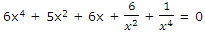# What is rational and integral polynomial

Publish On: 2019-03-18

Total Post: 542

# Mjay Jollay

Total Post: 0

## ANS: What is rational and integral polynomial

An expression of the form ƒ(x) = a0xn + a1xn-1 + …. + an-1 + an, where (i) a0, a1, …., an are constants, real or imaginary, (ii) x is a variable, and (iii) n is a positive integer, is called a rational integral function of x or a polynomial in x. If the coefficients a0, a1, a2, …., an are real number then the equation is called an equation with real coefficients.

Coefficients: The constants a0, a1, ….., an are called the coefficients of the polynomial of ƒ(x).

Degree of polynomial: If a0 ≠ 0, then the polynomial ƒ(x) is of degree n, i.e. it is the highest power of the variable x in the polynomial.

Identically vanishing polynomial: A polynomial all of whose coefficients are equal to zero is called an identically vanishing polynomial and is represented by 0, i.e. the polynomial a0xn + a1xn-1 + …. + an will be an identically vanishing polynomial if ai = 0, ∀ 1 ≤ i ≤ n.

Note 1: No degree is assigned to an identically vanishing polynomial.

Note 2: Constants (other than zero) are polynomial of degree zero.

Equality of polynomials: Two polynomials ƒ(x) = a0xn + a1xn-1 + …. + an, a0 ≠ 0 and g(x) = b0xm + b1xm-1 + …. + bm b0 ≠ 0, are said to be equal if

(i) They are of the same degree, i.e. m = n.

(ii) Their corresponding coefficients are equal, i.e. ai = bi, ∀ 1 ≤ i ≤ m.

Equation: If two different polynomials in the same variable x become equal for some values of x or a polynomial is equated to zero, then such a relation is called an equation, i.e. the polynomial  ƒ(x) = a0xn + a1xn-1 + …. + an, a0 ≠ 0, will be an equation if ƒ(x) = a0xn + a1xn-1 + …. + an, a0 = 0, for some values of x.

Degree of an equation: The degree of an equation is the highest power of the variable in the equation, when it is expressed as a rational and integral function of the variable.

For example, consider the following equations:x-3/2 + 4x2 = 3x1/2

The equation (i) when expressed in rational and integral form is 6x8 + 5x6 + 6x5 + 6x2 + 1 = 0 and thus its degree is 8 and the equation (ii) when expressed in rational integral form is of degree 3 for

9x3 – 6x2 + x – 16 = 0.

Note 3: The equation of degree two, three and four are called quadratic, cubic and biquadratic respectively.